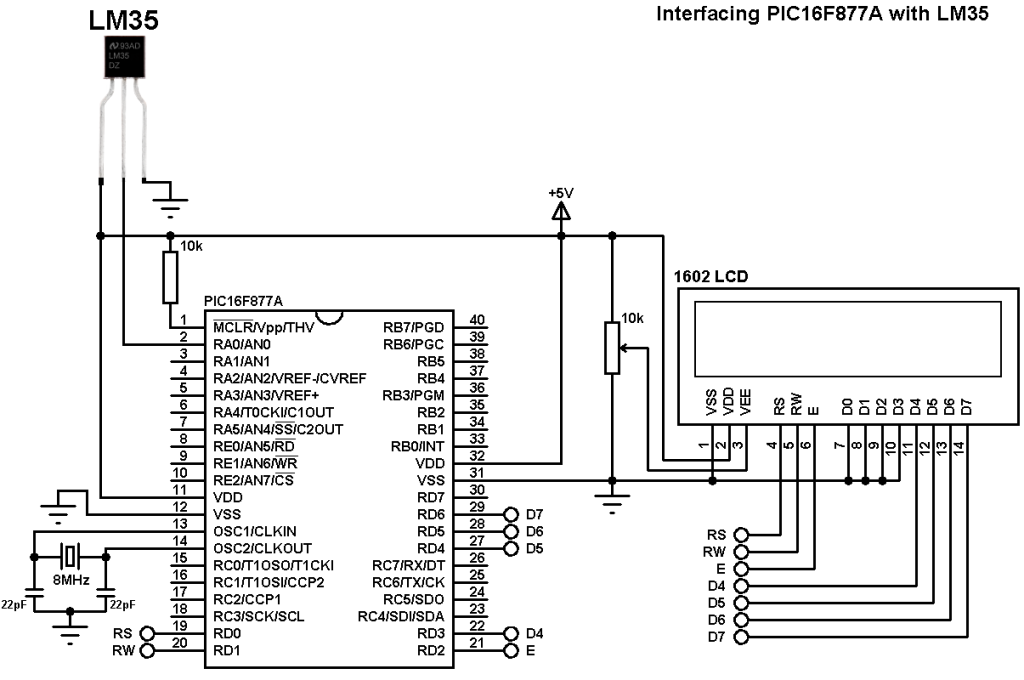# Interfacing PIC16F877A with LM35 temperature sensor – CCS C

The LM35 temperature sensor is three pin device (VCC, OUT and GND) with an output voltage linearly related to Centigrade temperature. Since the LM35 output varies with dependent to the temperature we need ADC (Analog-to-Digital Converter) module to measure this voltage. The ADC module converts analog data into digital data.
The LM35 output has linear +10mV/°C scale factor means the following:
If the output voltage =   10mV —> temperature =   1°C
If the output voltage = 100mV —> temperature = 10°C
If the output voltage = 200mV —> temperature = 20°C
If the output voltage = 370mV —> temperature = 37°C
and so on.

LM35 Futures (from datasheet):

• Calibrated Directly in ° Celsius (Centigrade)
• Linear + 10 mV/°C Scale Factor
• 0.5°C Ensured Accuracy (at +25°C)
• Rated for Full −55°C to +150°C Range
• Suitable for Remote Applications
• Low Cost Due to Wafer-Level Trimming
• Operates from 4 to 30 V
• Less than 60-μA Current Drain
• Low Self-Heating, 0.08°C in Still Air
• Nonlinearity Only ±¼°C Typical
• Low Impedance Output, 0.1 Ω for 1 mA Load

This topic shows how to interface the microcontroller PIC16F877A with LM35 analog temperature sensor.

Hardware Required:

• PIC16F877A microcontroller
• LM35 temperature sensor  — datasheet
• 1602 LCD screen
• 8MHz crystal
• 2 x 22pF ceramic capacitor
• 10K ohm variable resistor
• 5V voltage source
• Jumper wires

Interfacing PIC16F877A with LM35 sensor circuit:The output of the LM35 temperature sensor is connected to analog channel 0 (AN0) of the PIC16F877A.
In this example the microcontroller runs with crystal oscillator @ 8MHz.

Interfacing PIC16F877A with LM35 temperature sensor C code:
The C code below was tested with CCS PIC C compiler version 5.051.
Reading voltage quantity using the ADC gives us a number between 0 and 1023 (10-bit resolution), 0V is represented by 0 and 5V is represented by 1023. Converting back the ADC digital value is easy and we can use the following equation for that conversion: